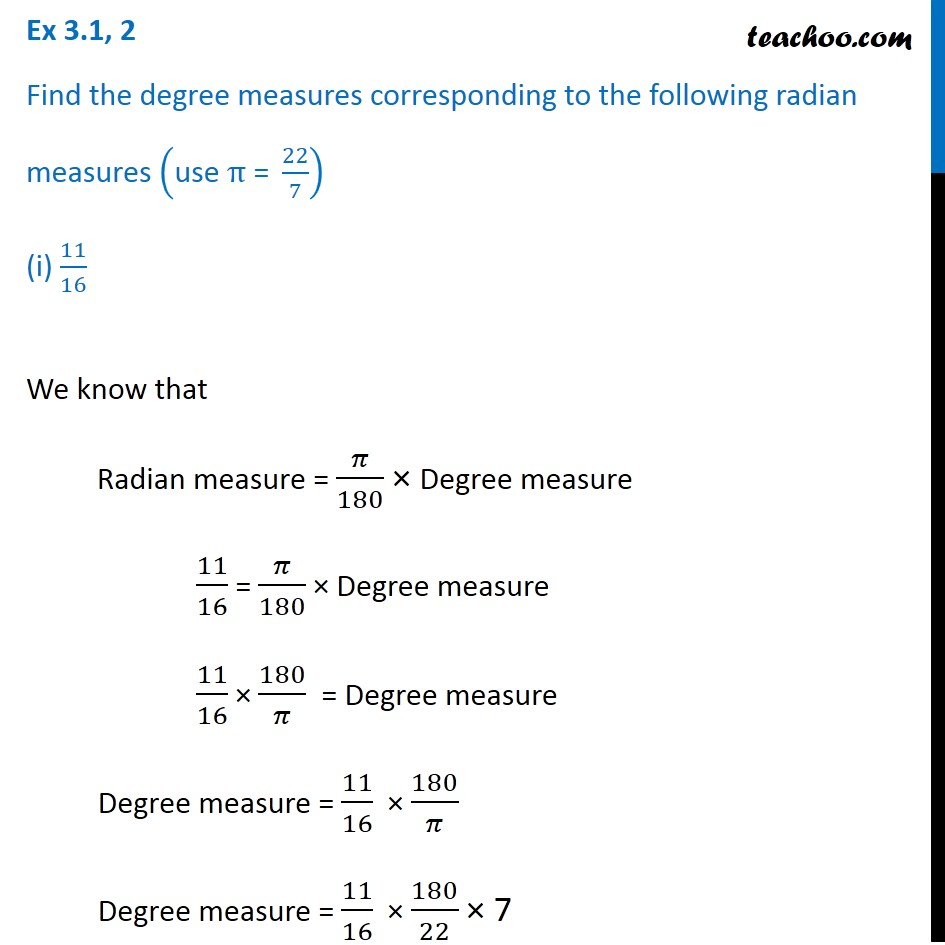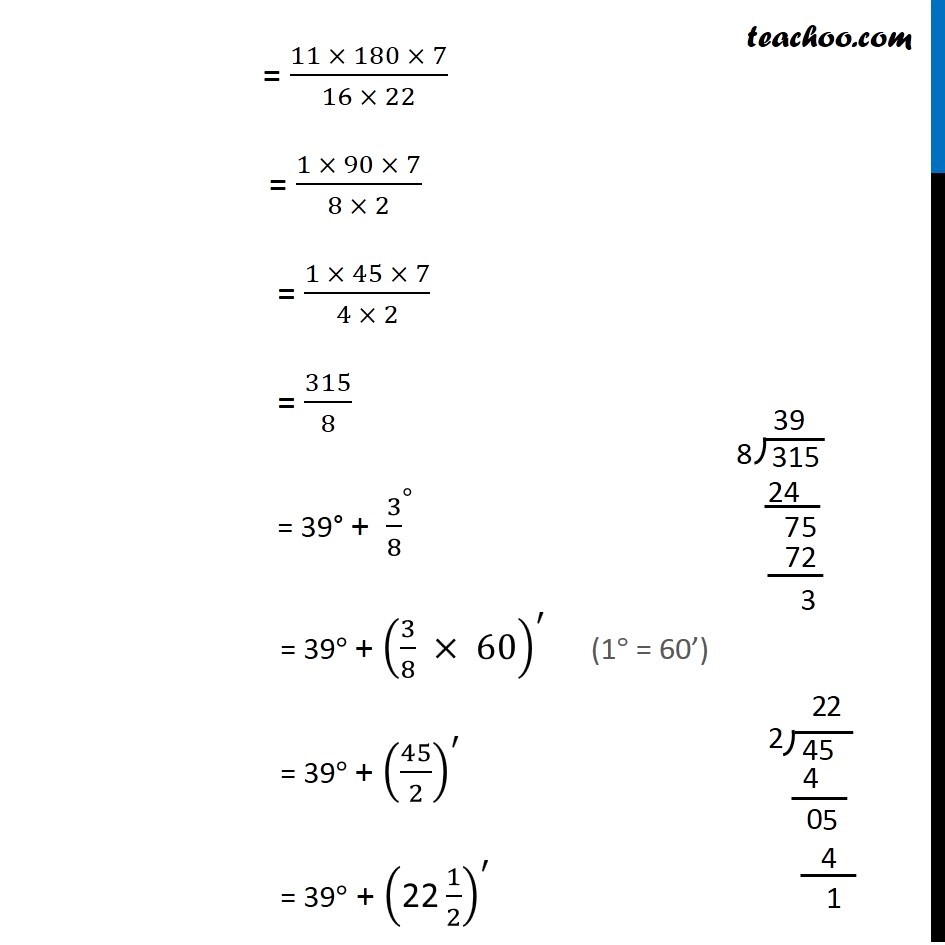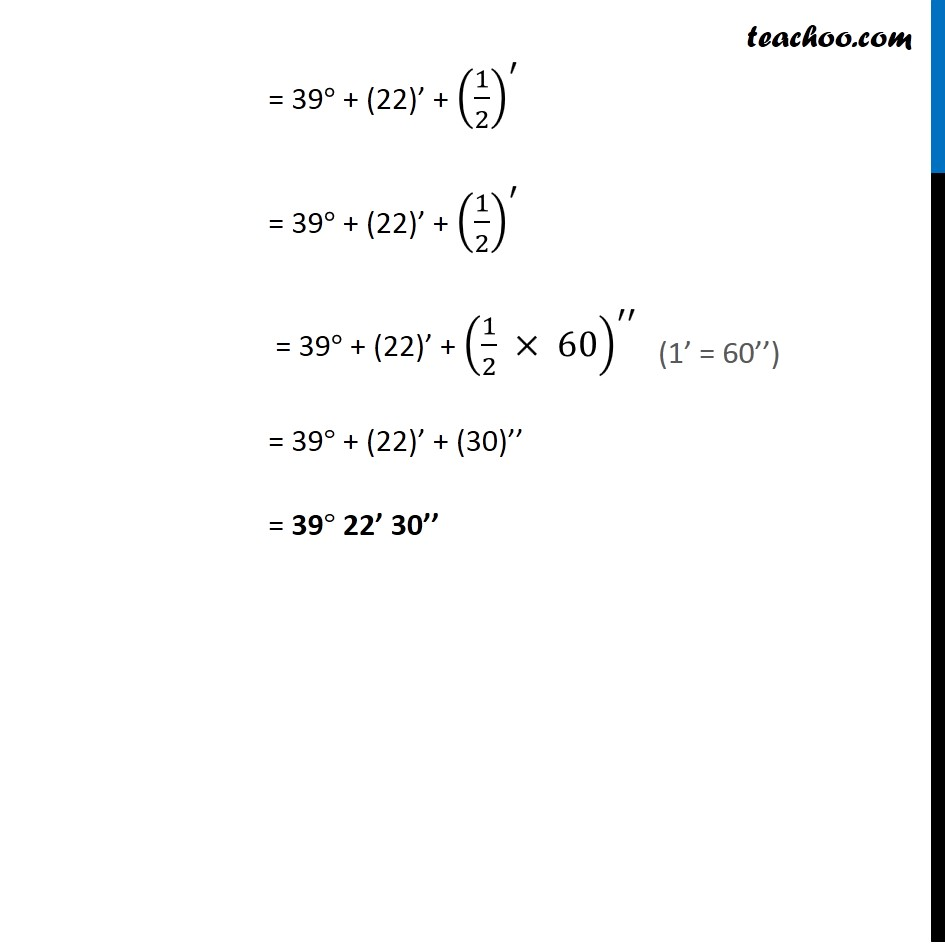1. Chapter 3 Class 11 Trigonometric Functions (Term 2)
2. Concept wise

Transcript

Ex 3.1, 2 Find the degree measures corresponding to the following radian measures ("use " π" = " 22/7) (i) 11/16 We know that Radian measure = 𝜋/180 × Degree measure 11/16 = 𝜋/180 × Degree measure 11/16 × 180/𝜋 = Degree measure Degree measure = 11/16 × 180/𝜋 Degree measure = 11/16 × 180/22 × 7 = (11 × 180 × 7)/(16 × 22) = (1 × 90 × 7)/(8 × 2) = (1 × 45 × 7)/(4 × 2) = 315/8 = 39° + 〖3/8〗^° = 39° + (3/8 × 60)^′ = 39° + (45/2)^′ = 39° + ("22" 1/2)^′ = 39° + (22)’ + (1/2)^′ = 39° + (22)’ + (1/2)^′ = 39° + (22)’ + (1/2 × 60)^′′ = 39° + (22)’ + (30)’’ = 39° 22’ 30’’